# Creating MNE objects from data arrays¶

In this simple example, the creation of MNE objects from numpy arrays is demonstrated. In the last example case, a NEO file format is used as a source for the data.

# Author: Jaakko Leppakangas <jaeilepp@student.jyu.fi>
#

import numpy as np
import neo

import mne

print(__doc__)


Create arbitrary data

sfreq = 1000  # Sampling frequency
times = np.arange(0, 10, 0.001)  # Use 10000 samples (10s)

sin = np.sin(times * 10)  # Multiplied by 10 for shorter cycles
cos = np.cos(times * 10)
sinX2 = sin * 2
cosX2 = cos * 2

# Numpy array of size 4 X 10000.
data = np.array([sin, cos, sinX2, cosX2])

# Definition of channel types and names.
ch_names = ['sin', 'cos', 'sinX2', 'cosX2']


Create an info object.

# It is also possible to use info from another raw object.
info = mne.create_info(ch_names=ch_names, sfreq=sfreq, ch_types=ch_types)


Create a dummy mne.io.RawArray object

raw = mne.io.RawArray(data, info)

# Scaling of the figure.
# For actual EEG/MEG data different scaling factors should be used.
scalings = {'mag': 2, 'grad': 2}

raw.plot(n_channels=4, scalings=scalings, title='Data from arrays',
show=True, block=True)

# It is also possible to auto-compute scalings
scalings = 'auto'  # Could also pass a dictionary with some value == 'auto'
raw.plot(n_channels=4, scalings=scalings, title='Auto-scaled Data from arrays',
show=True, block=True)

•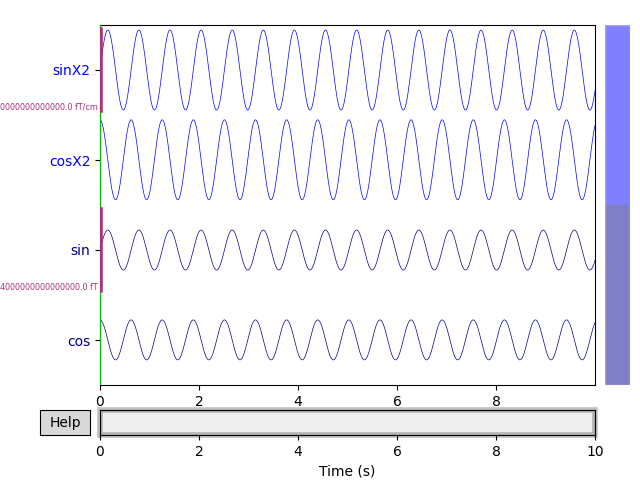•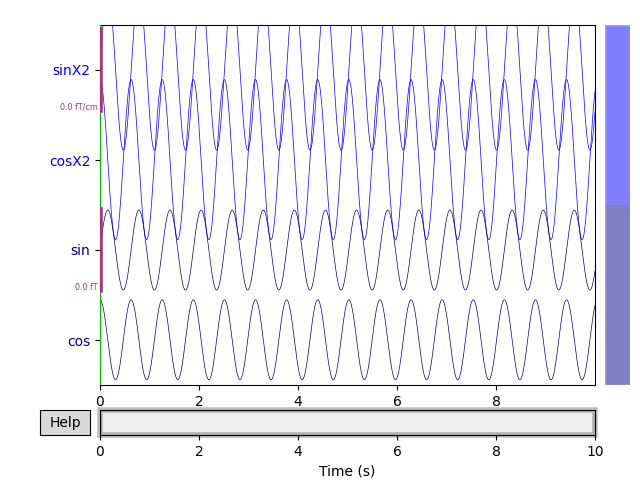Out:

Creating RawArray with float64 data, n_channels=4, n_times=10000
Range : 0 ... 9999 =      0.000 ...     9.999 secs


EpochsArray

event_id = 1  # This is used to identify the events.
# First column is for the sample number.
events = np.array([[200, 0, event_id],
[1200, 0, event_id],
[2000, 0, event_id]])  # List of three arbitrary events

# Here a data set of 700 ms epochs from 2 channels is
# created from sin and cos data.
# Any data in shape (n_epochs, n_channels, n_times) can be used.
epochs_data = np.array([[sin[:700], cos[:700]],
[sin[1000:1700], cos[1000:1700]],
[sin[1800:2500], cos[1800:2500]]])

ch_names = ['sin', 'cos']
ch_types = ['mag', 'mag']
info = mne.create_info(ch_names=ch_names, sfreq=sfreq, ch_types=ch_types)

epochs = mne.EpochsArray(epochs_data, info=info, events=events,
event_id={'arbitrary': 1})

picks = mne.pick_types(info, meg=True, eeg=False, misc=False)

epochs.plot(picks=picks, scalings='auto', show=True, block=True)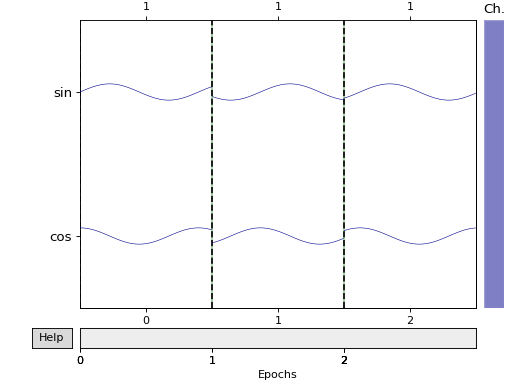Out:

Not setting metadata
3 matching events found
No baseline correction applied
0 projection items activated


EvokedArray

nave = len(epochs_data)  # Number of averaged epochs
evoked_data = np.mean(epochs_data, axis=0)

evokeds = mne.EvokedArray(evoked_data, info=info, tmin=-0.2,
comment='Arbitrary', nave=nave)
evokeds.plot(picks=picks, show=True, units={'mag': '-'},
titles={'mag': 'sin and cos averaged'}, time_unit='s')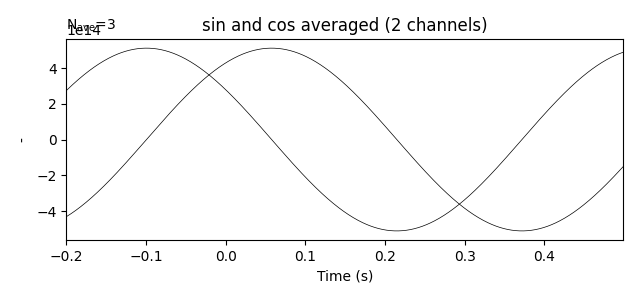Create epochs by windowing the raw data.

# The events are spaced evenly every 1 second.
duration = 1.

# create a fixed size events array
# start=0 and stop=None by default
events = mne.make_fixed_length_events(raw, event_id, duration=duration)
print(events)

# for fixed size events no start time before and after event
tmin = 0.
tmax = 0.99  # inclusive tmax, 1 second epochs

# create :class:Epochs <mne.Epochs> object
epochs = mne.Epochs(raw, events=events, event_id=event_id, tmin=tmin,
tmax=tmax, baseline=None, verbose=True)
epochs.plot(scalings='auto', block=True)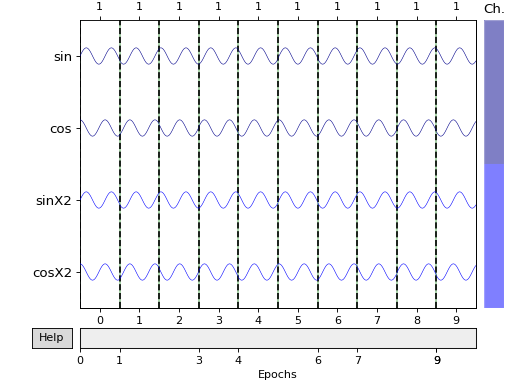Out:

[[   0    0    1]
[1000    0    1]
[2000    0    1]
[3000    0    1]
[4000    0    1]
[5000    0    1]
[6000    0    1]
[7000    0    1]
[8000    0    1]
[9000    0    1]]
10 matching events found
No baseline correction applied
0 projection items activated


Create overlapping epochs using mne.make_fixed_length_events() (50 % overlap). This also roughly doubles the amount of events compared to the previous event list.

duration = 0.5
events = mne.make_fixed_length_events(raw, event_id, duration=duration)
print(events)
epochs = mne.Epochs(raw, events=events, tmin=tmin, tmax=tmax, baseline=None,
verbose=True)
epochs.plot(scalings='auto', block=True)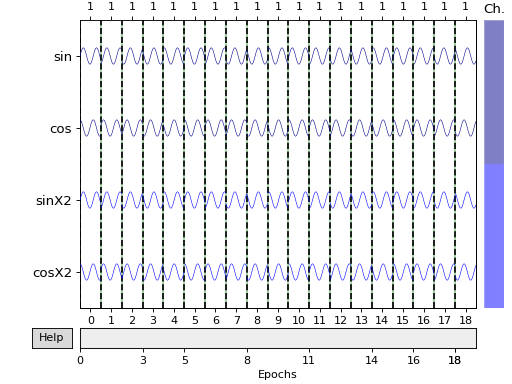Out:

[[   0    0    1]
[ 500    0    1]
[1000    0    1]
[1500    0    1]
[2000    0    1]
[2500    0    1]
[3000    0    1]
[3500    0    1]
[4000    0    1]
[4500    0    1]
[5000    0    1]
[5500    0    1]
[6000    0    1]
[6500    0    1]
[7000    0    1]
[7500    0    1]
[8000    0    1]
[8500    0    1]
[9000    0    1]
[9500    0    1]]
20 matching events found
No baseline correction applied
0 projection items activated


Extracting data from NEO file

# The example here uses the ExampleIO object for creating fake data.
# For actual data and different file formats, consult the NEO documentation.

# Get data from first (and only) segment
seg = bl.segments
title = seg.file_origin

ch_names = list()
data = list()
for ai, asig in enumerate(seg.analogsignals):
# Since the data does not contain channel names, channel indices are used.
ch_names.append('Neo %02d' % (ai + 1,))
# We need the ravel() here because Neo < 0.5 gave 1D, Neo 0.5 gives
# 2D (but still a single channel).
data.append(asig.rescale('V').magnitude.ravel())

data = np.array(data, float)

sfreq = int(seg.analogsignals.sampling_rate.magnitude)

# By default, the channel types are assumed to be 'misc'.
info = mne.create_info(ch_names=ch_names, sfreq=sfreq)

raw = mne.io.RawArray(data, info)
raw.plot(n_channels=4, scalings={'misc': 1}, title='Data from NEO',
show=True, block=True, clipping='clamp')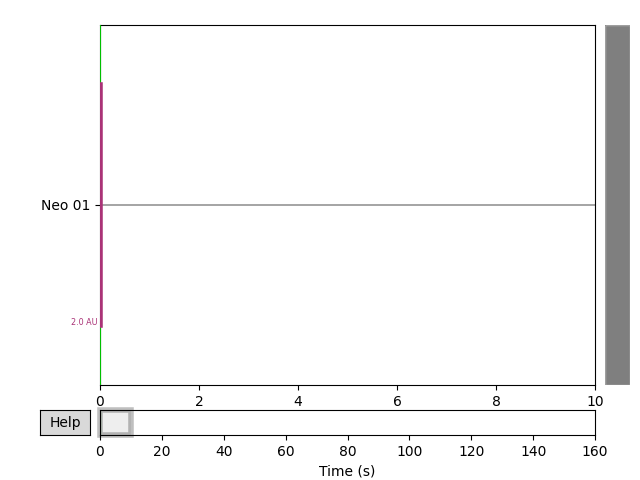Out:

Creating RawArray with float64 data, n_channels=1, n_times=1600000
Range : 0 ... 1599999 =      0.000 ...   160.000 secs